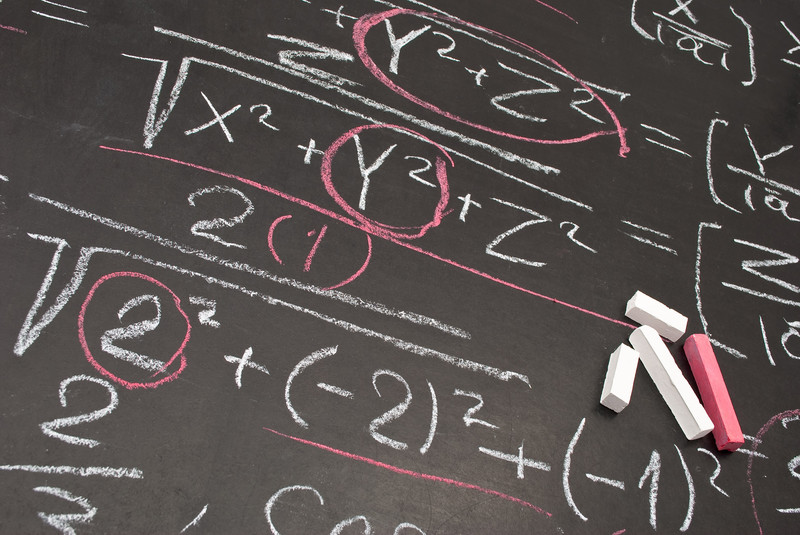# Algebraic Expressions and Equations

This post will focus mainly on expressions and their role in algebra. Expressions play a critical role in mathematics and we all have had to try and understand what they are as well as what they mean.Expression Defined

To understand what an expression is you first need to know what operation symbols are. Operation symbols tell you to do something to numbers or variables. Examples include the plus, minus, multiply, divide, etc.

An expression is a number, variable, or a combination of numbers[s] and variable[s] that use operation symbols. Below is an example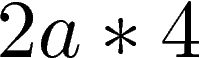Expressions consist of two terms and these are variables and constants. A variable is a letter that represents a number that can change. In our example above, the letter a is a variable.

A constant is a number whose value remains the same. In our example above, the numbers 2 and 4 are constants.

Expression vs Equation

An equation is when two expressions connected by an equal sign as shown below.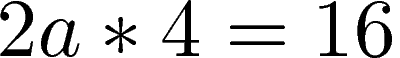In the example above we have to expressions. To the left of the equal sign is 2a * 4 and to the right of the equal sign is 16. Remember that an expression can be numbers and or variables so 16 is an expression because it is a number.

Simplify an Expression

Simplifying an expression involves completing as much math as possible to reduce the complexity of an expression. Below is an example.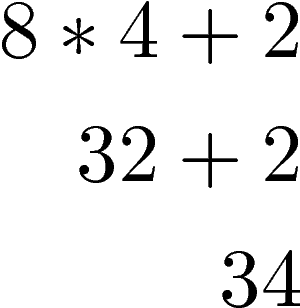In order to complete this expression  above you need to know the order of operations which is explained below

Parentheses
Exponents
Multiplication Division

In the example above, we begin with multiplication of 8 and 4 before we do the addition of adding 2. It’s important to remember that for multiplication/division or addition/subtraction that you move from left to right when dealing with these operation symbols in an expression. It is also important to know that subtraction and division are not associative (or commutative) that is: (1 – 2) – 3 != 1 – (2 – 3).

Evaluating an Expression

Evaluating an expression is finding the value of an expression when the variable is replaced with a specific number.Combining Like Terms

A common skill in algebra is the ability to combine like terms. A term is a constant or constant with one or more variables. Terms can include a constant such as 7 or a number and variable product such as 7a. The constant that multiplies the variable is called a coefficient. For example, 7a, 7 is the constant and the coefficient while a is the variable.

Combining like terms involves combining constant are variables that have the same characteristics for example

• 3 and 2 are like terms because they are both constants
• 2x and 3x are like terms because they are both constants with the same variable

Below is an example of combining like terms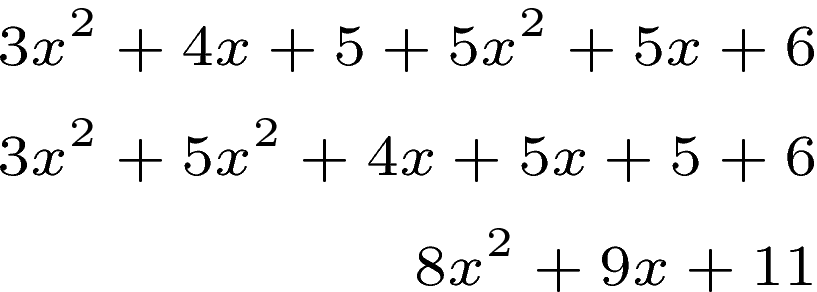In this example, we first placed like terms next to each other. This makes it easier to add them together. The rest is basic math.

Conclusion

Hopefully, the concept of expressions makes more sense. This is a foundational concept in mathematics that if you do not understand. It is difficult to go forward in the study of math.

## 1 thought on “Algebraic Expressions and Equations”

1.Denis-Pecheur Micheline

fine explanation of the basis of algebra .